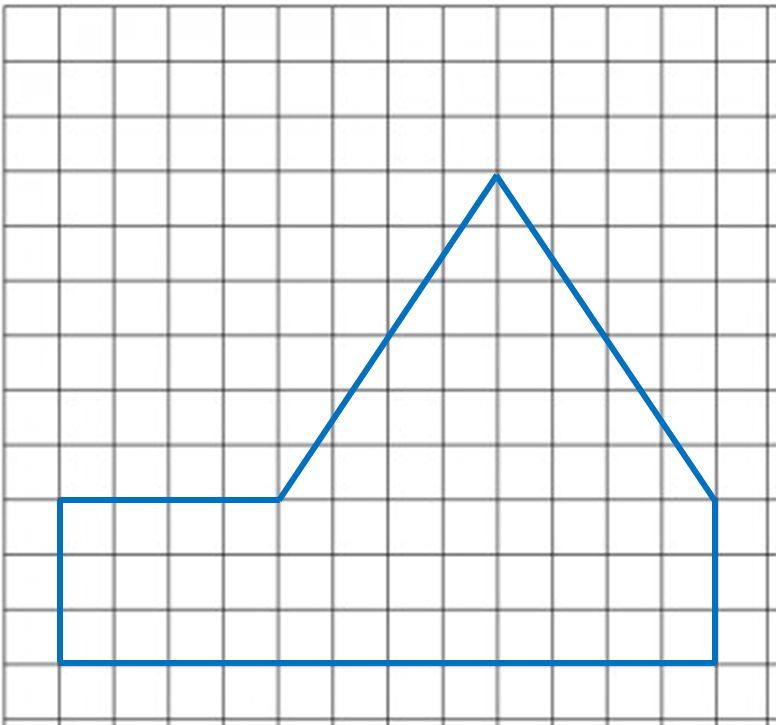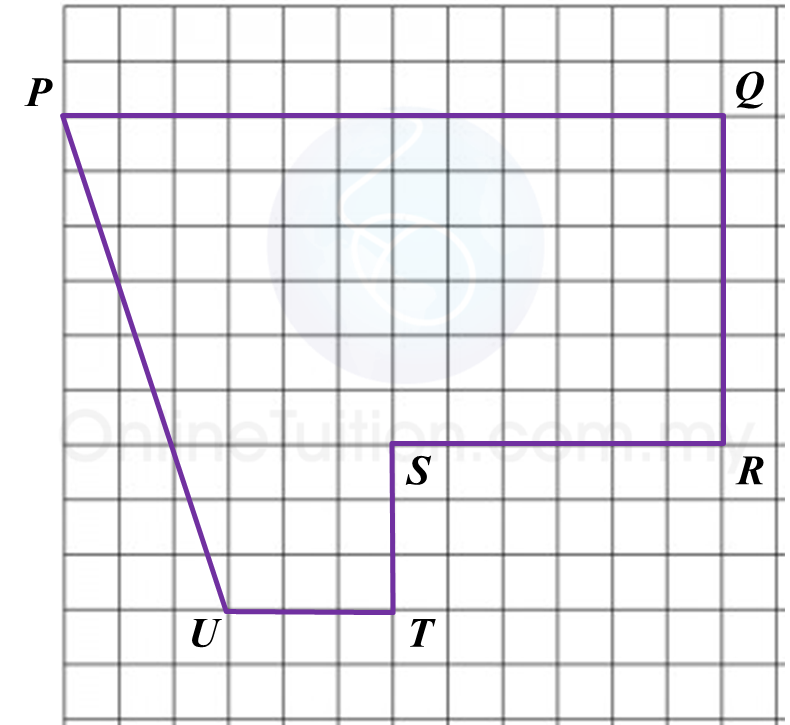# 4.2.2 Scale Drawings, PT3 Focus Practice

Question 4:
Diagram below shows polygon.(a) If the polygon is redrawn using the scale 1 : 500, calculate the length of side drawn for the side 15 m.
(b) On the square grids in the answer space, redraw the polygon using the scale 1 : 500. The grid has equal squares with sides of 1 cm.

(b)Question 5:
Diagram below shows a polygon PQRSTU.(a) If the polygon is redrawn using the scale 1 : 400, calculate the length of PQ.
(b) In the answer space, redraw the polygon using the scale 1 : 400. The grid has equal squares with sides of 1 cm.